Disclaimer: This is an example of a student written essay.

Any opinions, findings, conclusions or recommendations expressed in this material are those of the authors and do not necessarily reflect the views of UKEssays.com.

# An Explanation of the Fibonacci Sequence

 ✅ Paper Type: Free Essay ✅ Subject: Mathematics ✅ Wordcount: 3609 words ✅ Published: 5th Nov 2021

## Abstract

In this paper, I will examine the properties of Fibonacci Sequence and some identities related to Fibonacci sequence. I will also touch the applications of Fibonacci numbers, recurrence and closed forms of Fibonacci sequence.

## Contents

1 Introduction

2 Fibonacci Numbers in Pascal's Triangle

3 Cassini's Identity

4 Difference of Square of Fibonacci Numbers

5 Sum of Odd Fibonacci Numbers

6 Golden Ratio

7 Golden Spiral

8 Fibonacci Recurrence Relation

9 Fibonacci Closed Form

10 Conclusion

## 1 Introduction

Fibonacci numbers were discovered by Leonardo Pisano. His nickname is Fibonacci. The Fibonacci Sequence is the series of numbers:

0,1,1,2,3,5,8,13,21,34,...

Each number in the sequence is sum of the previous two terms.

u1 = 1 u2 = 1 un = un−1+un−2,n > 2

Fibonacci numbers first used in 'rabbit problem'. At the beginning, Fibonacci had one male and one female rabbits. He supposed that, every month. Next month more babies (male and female) were born. The next month these babies were grown and the first pair had two more babies (again male and female) . The next month the two adult pairs each have a pair of baby rabbits and the babies from last month mature. Fibonacci asked how many rabbits a single can produce after a year with this breeding process (rabbits never die, every month each adult pair produces a mixed pair of baby rabbits who mature the next month). Fibonacci asked how many would be formed in a year. Following the Fibonacci sequence perfectly the rabbits reproduction was determined 144 rabbits (Sinha,2017).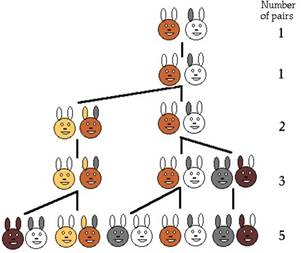Fig.1 rabbit problem diagram

## 2 Fibonacci Numbers in Pascal's Triangle

The Fibonacci Numbers are also applied in Pascal's Triangle. Entry is sum of the two numbers either side of it, but in the row above. Diagonal sums in Pascal's Triangle are the Fibonacci numbers.

An interesting property of Pascal's Triangle is that its diagonals sum to the Fibonacci sequence, as shown in the picture below: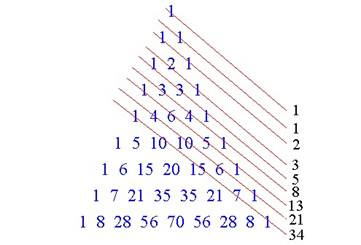Fig.2 diagonal sums in Pascal's triangle

The sum of the entries in the nth diagonal of Pascal's triangle is equal to the nth Fibonacci numbers for all positive integers n. Suppose ∑dn sum of the numbers at nth diagonal and fn = nth Fibonacci number, n ≥ 0.

Principle of Mathematical Induction will be used for proof.

For n = 0, ∑d0 = 0, f0 = 0 (The result is true for n = 0) Assume that ∑dk = fk fk+1 = fk+ fk1, Property of Fibonacci Sequence f(k) = ∑dk and f(k−1) = ∑dk1, inductive hypothesis

Therefore, ∑dk+1 = ∑dk+∑dk−1

F12+F22 = 12

## 3 Cassini's Identity

Cassini(1625-1712) is the astronomer who found Cassini Identity. Cassini's identity is a mathematical identity for Fibonacci sequence.

The history of science is silent as to why Cassini took such a great interest in Fibonacci numbers. Most likely it was simply a hobby of the Great astronomer. At that time many serious scientist took a great interest in Fibonacci numbers and the golden mean. These mathematical objects were also a hobby of Cassini's contemporary, Kepler(Stahbov,2012).

Fn+1Fn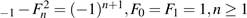### Proof by induction

Let's check that if the identity works for n=1.

For n = 1,2×1−12 = 1 = 11+1

Assume that the identity works for some n=k,

Fk+1Fk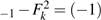k+1

We want to show that also

Fk+2Fk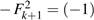k+2

To prove this we will establish

Fk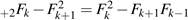Here is how:

Fk2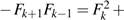FkFk−1−FkFk−1−Fk+1Fk−1

= Fk+1FkFkFkFk−1−Fk+1Fk−1

= Fk+1Fk−2FkFk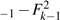(Fk+1+Fk)Fk−(Fk+Fk−1)2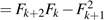## 4 Difference of Square of Fibonacci Numbers

The difference of the square of two consecutive Fibonacci numbers is equal to the product of two Fibonacci numbers.

F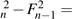FnFn−1

### Proof

Use the following identities.

F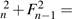FnFn−1

Fn+1 = Fn+Fn−1

an = F2n−1 bn = 2FnFn−1

cn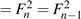a2n = b2n+c2n

F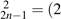FnFn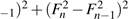F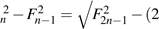FnFn−1)2

### Consider separately

F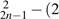FnFn−1)2.

F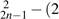FnFn−1)2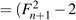FnFn−1)2−(2FnFn−1)2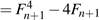FnFn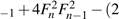FnFn−1)2

F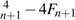FnFn−1

= (Fn+Fn−1)4−4(Fn+Fn−1)FnFn−1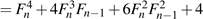FnF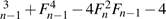FnFn2−1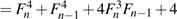FnF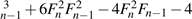FnFn2−1

After simplifying this by using identities

We can show that F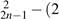FnFn1)2 = (FnFn1)2

Hence F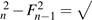(FnFn−1)2 So, F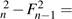FnFn−1.

## 5 Sum of Odd Fibonacci Numbers

The sum of odd Fibonacci numbers is also a Fibonacci number.

F1+F3+F5+...+F2n−1 = F2n,F0 = 0,F1 = 1

Proof by induction. base case: for n=1 F1 = 1 = F2 This proves that the base case is true.

inductive step: Lets assume that it is true for some n ≥ 1.

F1+F3+F5+...+F2n−1 = F2n

We need to prove that it is also true for n+1.

F1+F3+F5+...+F2(n+1)−1 = (F1+F3+F5+...+F2n−1)+F2(n+1)−1

= F2n+F2(n+1)−1

= F2n+F2n+1

= F2n+2

= F2(n+1)

This proves that it is true for n+1. Hence it is true for all n ≥ 1.

## 6 Golden Ratio

Any Fibonacci number divided by the previous Fibonacci number has a quotient of approximately 1.618034...

FFnn1 ≈ 1.618034(φ)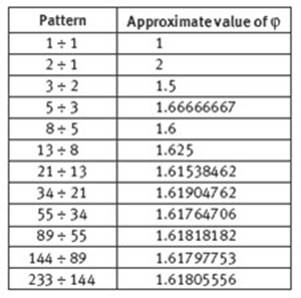Fig.3 ratio of consecutive Fibonacci numbers

The Golden Ratio is also found all throughout the physical universe. Weather patterns, Whirlpool have almost the same form like the golden spiral. Even the Sea Wave sometimes shows almost the same spiral pattern. The three rings of Saturn are designed naturally based on the Golden Ratio. The Galaxy, Milky Way, also has the spiral pattern almost like golden spiral. Relative planetary distances of the Solar System also have the golden ratio properties. (2011)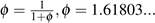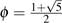There is a good change of variable analysis in textbook 'An Introduction to the Analysis of Algorithms' (2013).

an = 1/(1+an1) (2013)

Let's write some terms.

a0 = 1

a1 =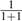=a2 = 1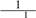+1+1 = 1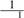+2=When we continue, we will see the pattern to continued fractions continues.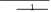a3 = 1+1+1 1 = 1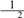+3=1+1

a4 =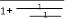1+1 = 1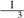+5=1+ 1

1+1

The form an = bbnn1 is certainly suggested:

substituting this equation into the recurrences gives

bbn1 = 1/(1+ bbnn21) for n > 1 with b0 = b1 = 1.

n

Dividing both sides by bn1gives

bn−1+1bn−2 for n > 1 with b0 = b1 = 1,

which implies that bn = Fn+1, the Fibonacci sequence. This argument generalizes to give a way to express general classes of "continues fraction" representations as solutions to recurrences (2013).

## 7 Golden Spiral

Golden spiral is derived from golden ratio.The growth factor of golden spiral is φ , the golden ratio.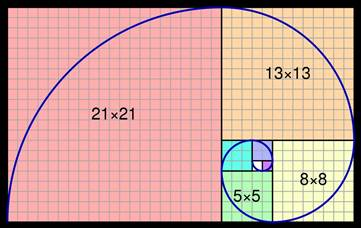Fig.4 golden spiral

To draw Golden Spiral, draw squares by using Fibonacci sequence. This works by drawing squares which have the side lengths of Fibonacci numbers and combining them.

Golden spiral is used in real life in many areas like art, beauty, clothing, architecture,logos etc.

The Golden ratio is prevalent in Da Vinci's The Annunciation, Madonna with Child and Saints, The Mona Lisa and St. Jerome. He was famous for using the Golden ratio in his works. The Mona Lisa, a well known portrait of a woman with a coy smile, is embedded with Golden rectangles (2018).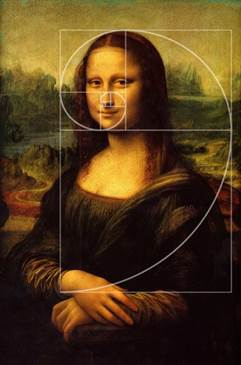Fig.5 The Mona Lisa

## 8 Fibonacci Recurrence Relation

We can find recurrence formula of Fibonacci sequence by using its definition.

Fn = Fn−1+Fn−2 xn = xn−1+xn−2

We will divide each term by xn−2

x2 = x+1 x2x−1 = 0

x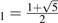x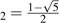Fn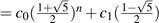n

F0 = 0,F1 = 1

c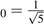c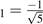Fn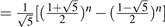Fibonacci Recurrence Relation

## 9 Fibonacci Closed Form

Let G(z) be closed form of Fibonacci sequence.

G(z) = F0+F1z+F2z2+F3z3+F4z4+...

Now let's find zG(z) and z2G(z) by multiplying G(z) by z and z2.

zG(z) = F0z+F1z2+F2z3+F3z4+F4z5+... z2G(z) = F0z2+F1z3+F2z4+F3z5+F4z6+...

Now we will find the difference of G(z)−zG(z)−z2G(z)

(1−zz2)G(z) = F0+(F1F0)z+(F2F1F0)z2+(F3F2F1)z3+...

We know that F1 = F2 = 1

so

F2F1 = 0.

Also since for n > 2 we have that

Fn = Fn−1+Fn−2 then

F3F2F1 = 0,F4F3F2 = 0,...,

Fn+1−FnFn−1 = 0.

Therefore:

(1−zz2)G(z) = z

This will give us the closed form of Fibonacci sequence as:

G(z) = 1−zzz2

## 10 Conclusion

In conclusion, studying Fibonacci numbers was great experience. It was interesting and joyful to see the harmony of numbers and the beauty they made. I searched the history of Fibonacci numbers, some identities related to Fibonacci numbers and the applications of Fibonacci numbers. Seeing the applications of Fibonacci numbers was eye opening.

## References

1. Sinha, Sudipta, 2017, The Fibonacci Numbers and Its Amazing Applications, International Journal of Engineering Science Invention, Volume 6, Issue6

2. Stakhov, Alexey, 2012, A generalization of the Cassini Formula, The International Clubof the Golden Section

3. Akhtaruzzaman, Md , Shafie, Amir A. ,2011, Geometrical Substantiation of Phi, theGolden Ratio and the Baroque of Nature, Architecture, Design and Engineering International Journal of Arts

4. Thapa ,Gyan Bahadur , Thapa , Rena, 2018, Journal of the Institute of Engineering, TheRelation of Golden Ratio, Mathematics and Aesthetics

5. Sedgewick, R., & Flajolet, P. (2013). An introduction to the analysis of Algorithms, (Second Edition). Addision-Wesley Professional.

6. Wiki page Golden Ratio. https://en.wikipedia.org/wiki/Goldenspiral

7. Fig.1 Knott, Ron, 2016, Fibonacci Numbers and Nature. http://www.maths.surrey.ac.uk/hostedsites/R.Knott/Fibonacci/fibnat.html

8. Fig.2 https://www.maplesoft.com/applications/view.aspx?SID=3617view=html

9. Fig.3 Ancient Architecture(ratio of consecutive Fibonacci numbers). https://nzmaths.co.nz/resource/anciarchitecture

10. Fig.4. Wikimedia commons. https://commons.wikimedia.org/wiki/File:FibonacciSpiral.svg

11. Fig.5. The Mona Lisa https://thefibonaccisequence.weebly.com/mona-lisa.html

View all

## DMCA / Removal Request

If you are the original writer of this essay and no longer wish to have your work published on UKEssays.com then please:

Related Services

Prices from

SR571

Approximate costs for:

• 1000 words
• 7 day deliveryHumanity University

Dedicated to your worth and value as a human being!

Related Lectures